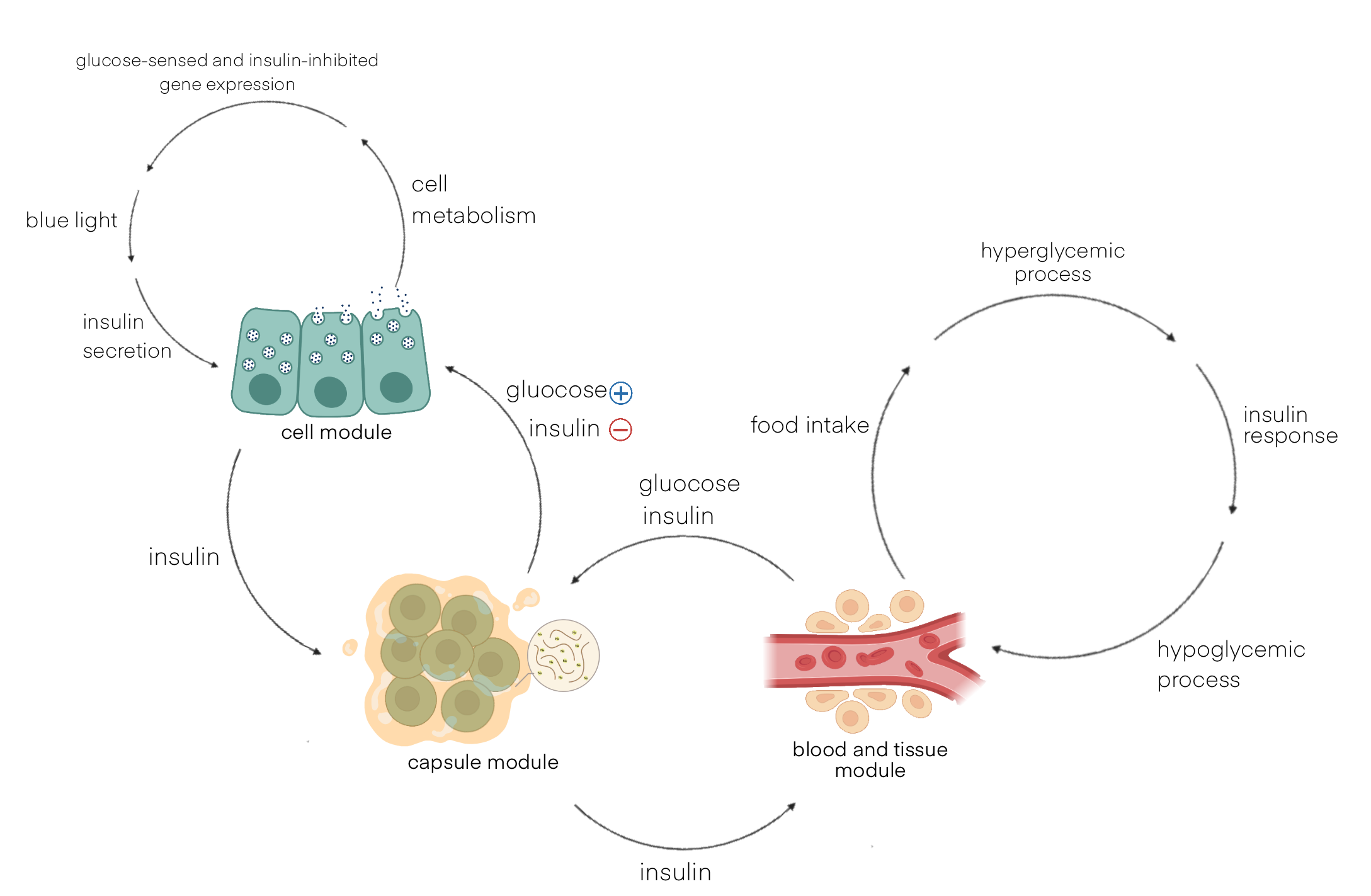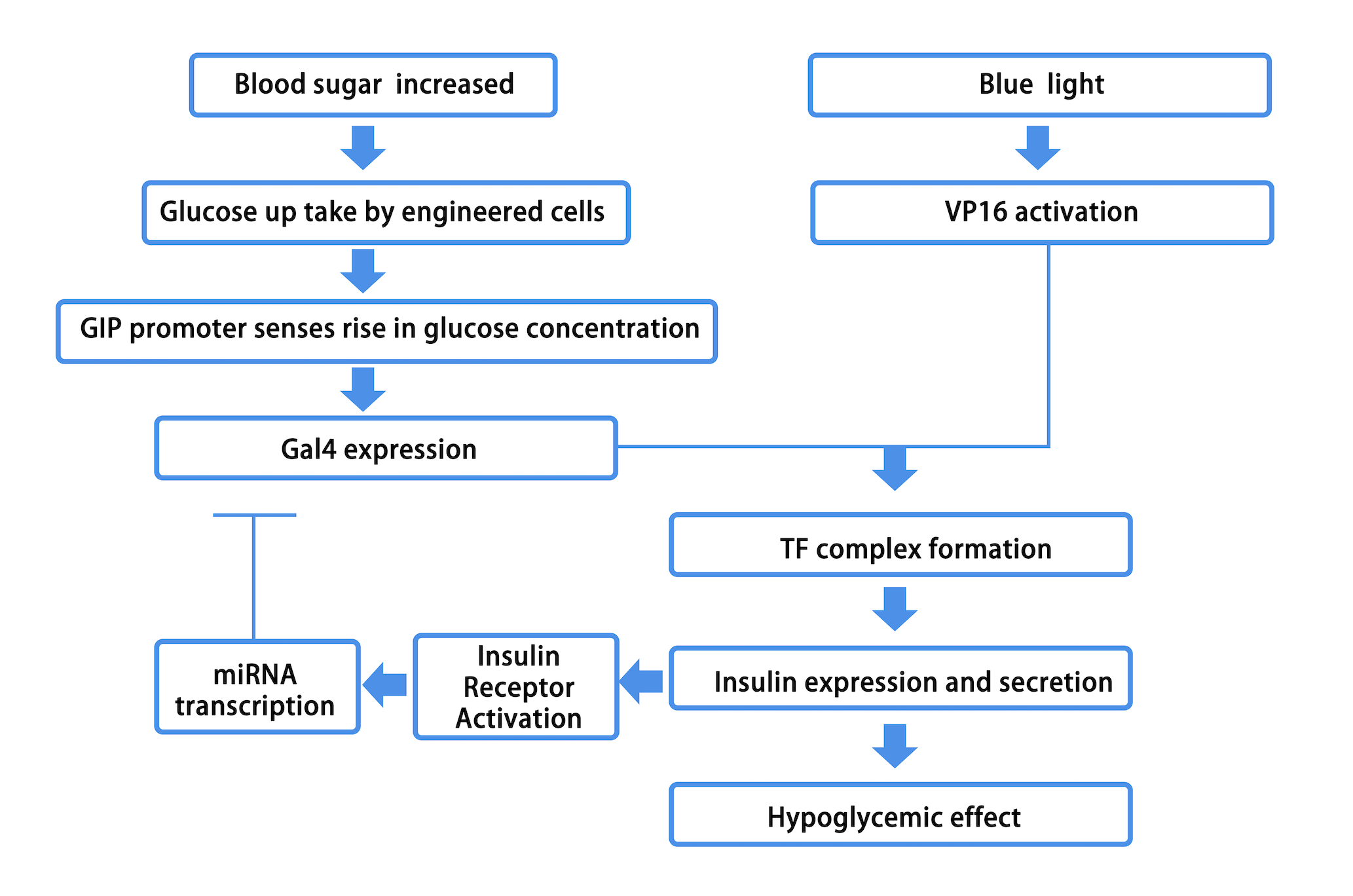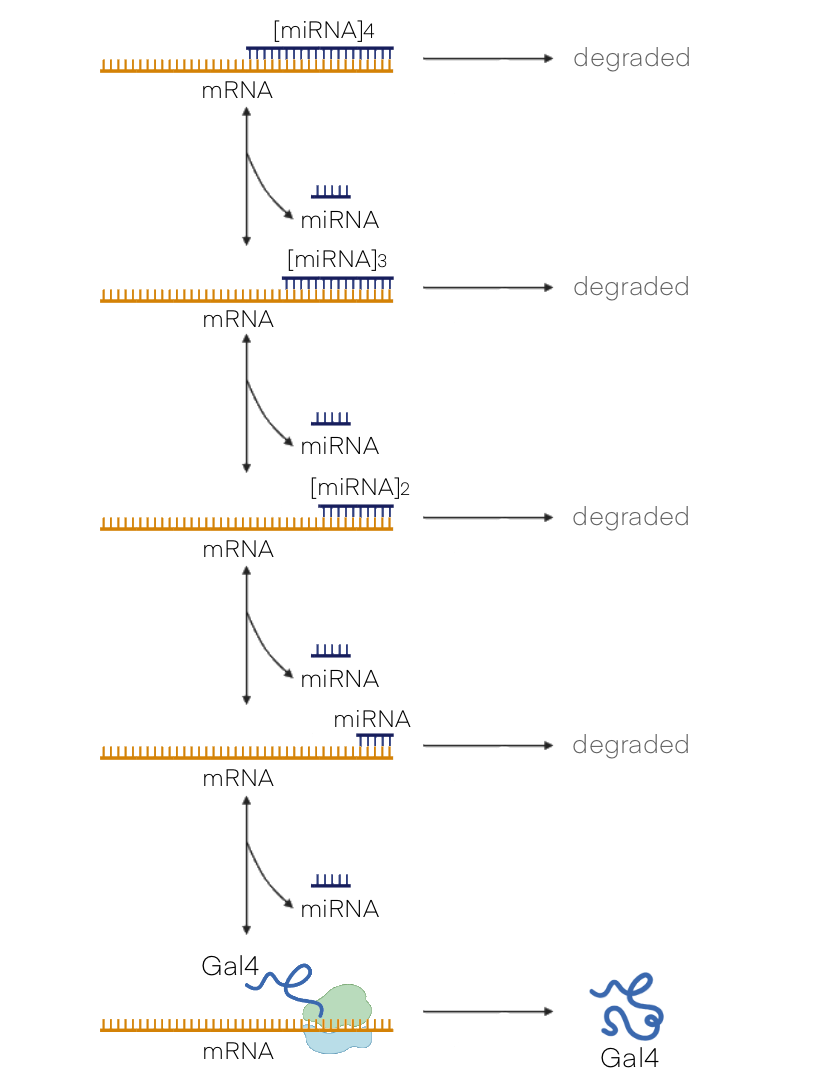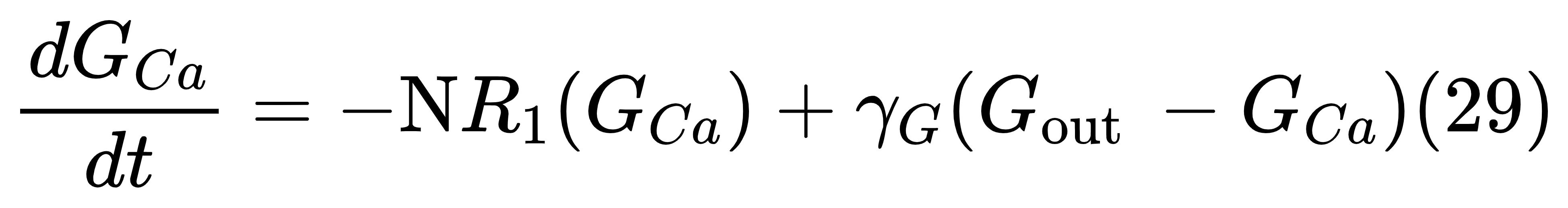Team:csu_china

# Dynamic mathematical model of Sweet Guard

## Construction of ODEs Model to Characterize Gene Circuit

To quantitatively analyze the workflow of Sweet Guard, ensure the consistency of the step design, verify the experimental data, predict the product operation and make further improvement of the product, we developed a dynamic mathematical model. This model is mainly constructed by ODE, which is based on the existing data. It simulates the gene circuit of engineered cells that sense blood glucose and blue light, followed by insulin secretion and the main physiological processes associated with it.

We have improved the existing model to make it suitable for our Sweet Guard design. The ODEs model is mainly composed of three modules:

1. Engineering cells. This module mainly includes the process that glucose enters the engineering cells to change the metabolic state of the cells, which leads to a series of responses and initiates the expression and secretion of insulin after blue light irradiation, and the process of negative feedback inhibition of their insulin expression after the engineering cells sense extracellular insulin.
2. Capsule. The sodium alginate matrix where the engineering cells are located. This module includes the process of proliferation of engineered cells and the diffusion of substances such as glucose and insulin.
3. Blood and tissue. It refers to the environment of blood and tissue metabolism throughout the body. This module simulates the dynamic changes of blood glucose in the body and the body's response to insulin.Figure1. Structure of the mathematical model.

## Detailed description

Due to space limitations, the detailed description of the model will be briefly introduced in the selection of modules, instead of explaining every equation. The specific parameters can be downloaded in pdf to view. For detailed information, please refer to the references of our model.

The equations used in ODEs mainly include the chemical master equation, the Hill equation, and the Michaelis equation. The chemical master equation mainly characterizes the mutual transformation between various molecules; the Hill equation is used to characterize the degree of activation of a substance that is concentration-dependent on another substance; the Michaelis equation mainly simulates the instantaneous rate of a certain process with the maximum rate under specific conditions.Figure2. Engineered cell gene expression process

### Cell module

We assume that when glucose enters the engineering cell, the entire circuit starts. The glucose concentration in the capsule is $G_{Ca}$, and it enters the cell at a rate of $NR_1$, where $N$ is the total number of engineered cells, and $R_1$ is the rate of glucose uptake by a single cell. The concentration of glucose in a single cell is Gin, and it is metabolically consumed at the rate of $R_2$ to generate intermediate products and $ATP$ (to simplify the equation, we "black-box" the complex sugar metabolism process into two steps: intermediate product generation and $ATP$ generation), $R_2$ depends on $ATP$ and intracellular glucose levels.The glucose metabolism intermediate product is the signal to activate the subsequent process, that is, the activation degree of the transcription activator of the Gal4 gene depends on the concentration of the intermediate product $XG$. The activated transcription activator transcribes the $Gal4$ gene to generate $Gal4\ mRNA$, which is translated into $Gal4$. Engineering cells produce $VP16$ constitutively, and their mRNA and protein concentrations are set to $MVP16$ and $PVP16$, respectively. $VP16$ itself is unactivated, and the radiation of blue light can quickly transform it into $VP16*$ of another configuration before it can reversibly bind with $Gal4$ to become the transcription factor $TF$ of the insulin gene. The $VP16$ of active configuration will be converted to the original inactive configuration by hydrolysis. $TF$ transcribes $insulin mRNA$.There are insulin receptors on the surface of engineering cells, the concentration of which is set to $P_{IR}$, the insulin in the matrix (see Capsule module for details) can activate the receptors, and the receptor-ligand complex ${P_{IR}}^*$ will also be hydrolyzed into individual receptors and ligands. For the sake of simplicity, here we also "black-box" the signal transduction process of the insulin receptor. After the insulin receptor-ligand complex is internalized, it acts as a signal molecule to catalyze the activation of the constitutively expressed $miRNA$ transcription factor.The activated $miRNA$ transcribes $miRNA$. $miRNA$ can reversibly bind to Gal4 mRNA, and because the latter has four miR-Targets, one $Gal4\ mRNA$ can bind up to 4 $miRNAs$. The $mRNA$ bound with $miRNA$ is easily degraded, and the rate of this reaction increases with the number of $miRNA$ rising.Figure3. Schematic representation of binding of Gal4 mRNA and miRNA### Capsule Module

After $TF$ transcribes insulin $mRNA$, the latter is translated into insulin and secreted into the sodium alginate capsule matrix. The concentration of insulin in sodium alginate is set as $P_{INS}$, and it diffuses between the substrate and the blood driven by the concentration gradient.In addition, glucose also needs to diffuse into the matrix before entering the engineering cells.### Blood and tissue module

This part of the model simulates the changes in blood glucose and insulin levels in the body under physiological and pathological conditions. When combined with the above two modules, the kinetic process of systemic blood glucose and insulin can be analyzed when Sweet Guard is running. Based on the analysis of existing data and our subsequent improvements, some notations in this module are as followed:

At the same time, we included an additional (virtual) intermediary compartment for glucose to allow for the adequate representation of dynamics in high intake of glucose in a short time, with a corresponding glucose concentration.

The core idea of this part is to use a proportional-integral-derivative (PID) controller to characterize the role of insulin (see references for details), and obtain equations about three insulin components:Here, the parameters $k_{iar}$ and $k_{iir}$ respectively represent the clearance constants of insulin in each component. The three terms in (32) represent insulin production proportional to the currentglucose concentration，proportional to the integral of previous deviations from the glucoseset-point, and proportional to the rate of change of the glucose concentration, respectively. For patients with type I diabetes, $F(·)=0$. The auxiliary function (33) can optimize (32), which can prevent the insulin level from becoming negative. Finally, the glucose concentration in blood sugar and additional components are as follows: